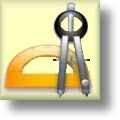Geometry Worksheets

Geometry Worksheets for Practice and Study

Here is a graphic preview for all of the Geometry Worksheets Sections. You can select different variables to customize these Geometry Worksheets for your needs. The Geometry Worksheets are randomly created and will never repeat so you have an endless supply of quality Geometry Worksheets to use in the classroom or at home. These Geometry Worksheets consist of Angle Worksheets, Circles Worksheets, Coordinate Geometry Worksheets, Quadrilaterals and Polygons Worksheets, Perimeter and Area Worksheets, Pythagorean Theorem Worksheets, Surface Area and Volume Worksheets, Triangle Worksheets, and Trigonometry Worksheets for your use. Our Geometry Worksheets are free to download, easy to use, and very flexible.

These Geometry Worksheets are a great resource for children in the 4th Grade, and 5th Grade, 6th Grade, 7th Grade, and 8th Grade.

Click here for a Detailed Description of all the Geometry Worksheet Sections.

Quick Link for All Geometry Worksheet Sections

Click the image to be taken to that Geometry Worksheet Section.

Angles WorksheetsGeometry Worksheets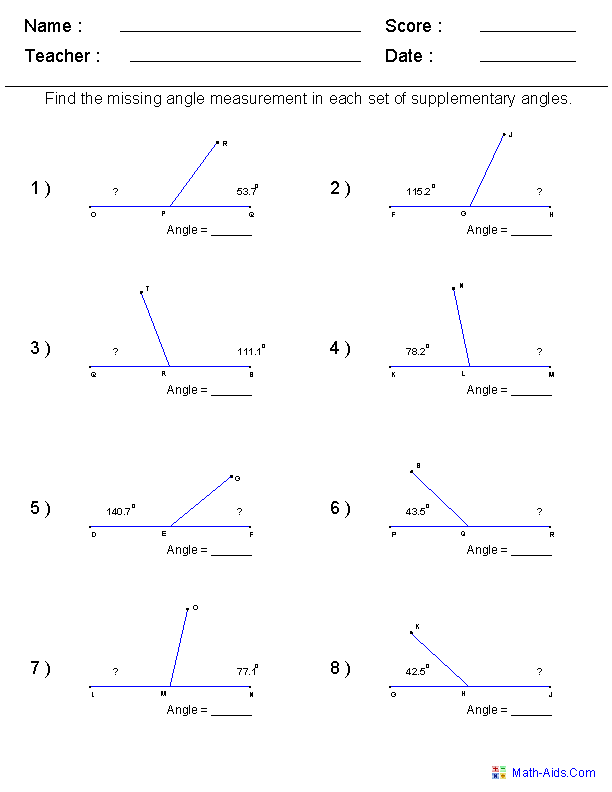Area and PerimeterGeometry WorksheetsCircle WorksheetsGeometry WorksheetsCoordinate GeometryGeometry Worksheets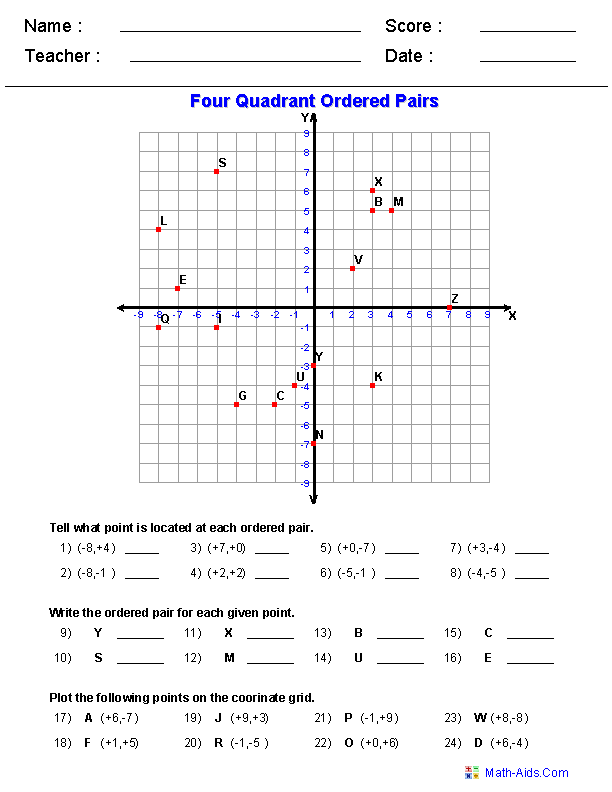Constructions WorksheetsGeometry WorksheetsQuadrilaterals & PolygonsGeometry Worksheets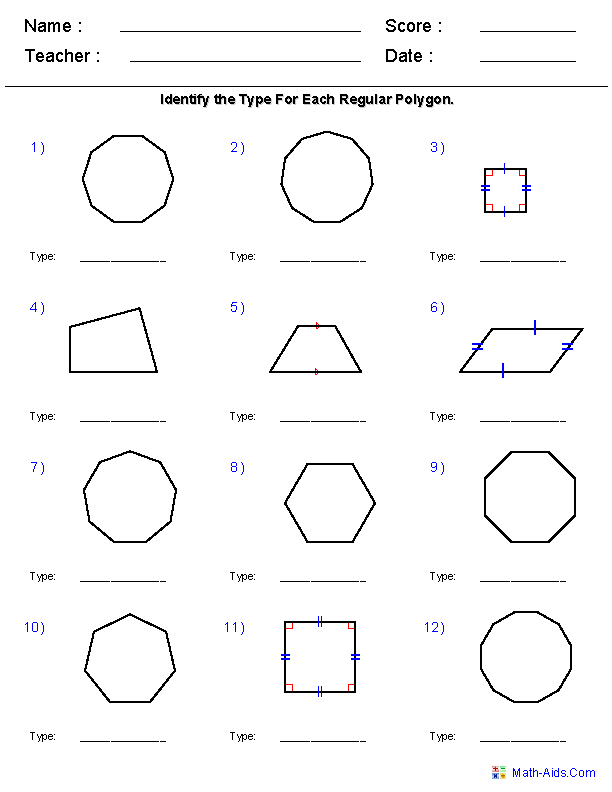Parallel and PerpendicularLines WorksheetsPythagorean TheoremGeometry Worksheets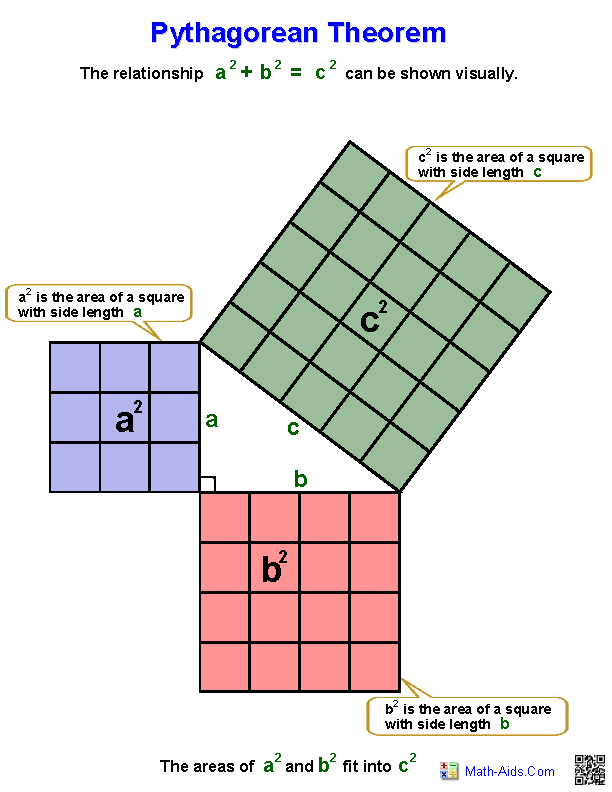SimilarityGeometry Worksheets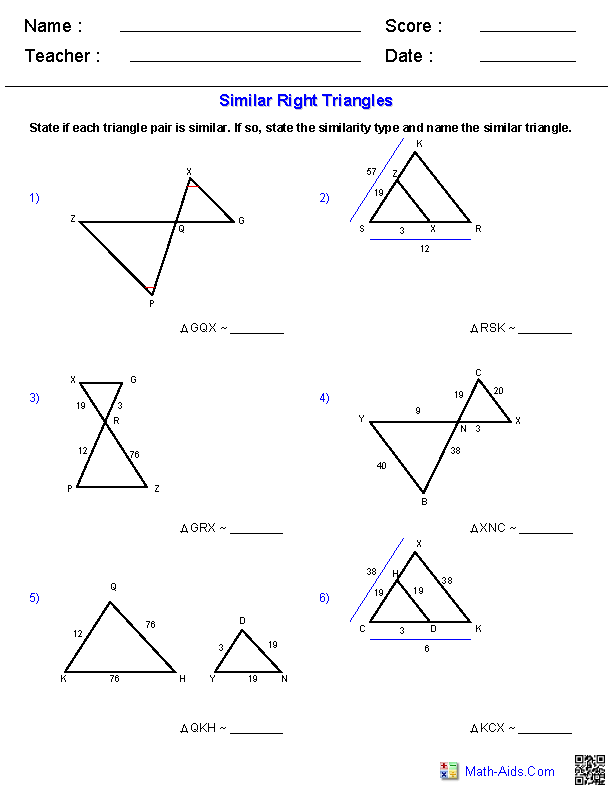Surface Area & VolumeGeometry Worksheets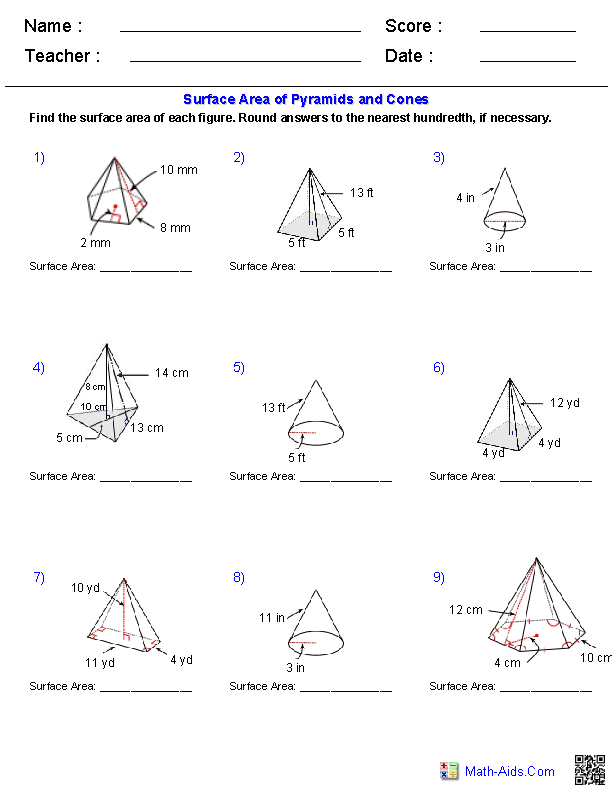Transformation WorksheetsGeometry Worksheets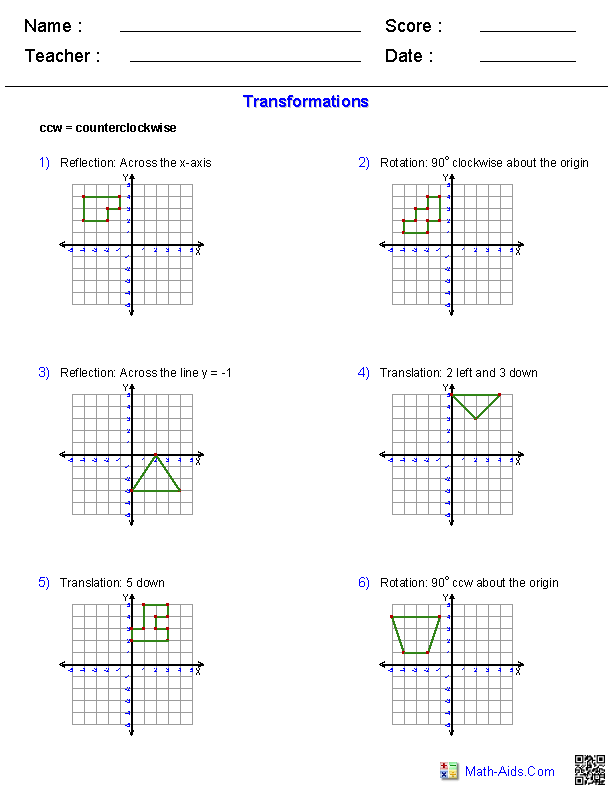Triangle WorksheetsGeometry Worksheets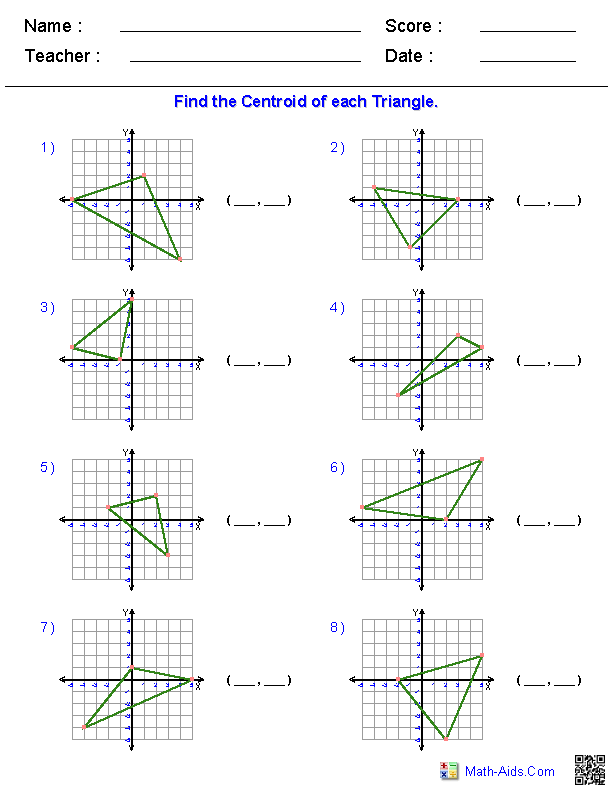Trigonometry WorksheetsGeometry WorksheetsRecommended Videos

Detailed Description for All Geometry Worksheet Sections

Angles Worksheets
This section contains all of the graphic previews for the Angles Worksheets. We have classifying, naming, and measuring angles worksheets, reading protractors worksheets, finding complementary, supplementary, vertical, alternate, corresponding angles and much more angle worksheet for your use. These geometry worksheets are a good resource for children in the 5th Grade through the 10th Grade.

Area and Perimeter Worksheets
This section contains all of the graphic previews for the Area and Perimeter Worksheets. We have area and perimeter worksheets for triangles, rectangles, parallelograms, trapezoids, regular polygons, quadrilaterals, and a formula worksheet for your use. These geometry worksheets are a good resource for children in the 5th Grade through the 10th Grade.

Circle Worksheets
This section contains all of the graphic previews for the Circle Worksheets. We have identifying radius and diameter for circles worksheets, calculating circumference, area, radius, and diameters worksheets, arcs and central angles for circles worksheets, arcs and chords worksheets, inscribed angles worksheets, graphing of circles worksheets and much more circle worksheets for your use. These geometry worksheets are a good resource for children in the 5th Grade through the 10th Grade.

Constructions Worksheets
This section contains all of the graphic previews for the Constructions Worksheets. We have constructing line segments, perpendicular segments, angles, triangles, median of triangles, altitudes of triangles, angle bisectors, and circles. These geometry worksheets are a good resource for children in the 5th Grade through the 10th Grade.

Coordinate Worksheets
This section contains all of the graphic previews for the Coordinate Worksheets. We have identifying lines, rays and line segments worksheets, measurement of line segments worksheets, lines on a coordinate plane worksheets, midpoint formula worksheets, distance formula worksheets, translation, rotation, and reflection worksheets, single and four quadrant ordered pair worksheets, four quadrant graphing puzzle worksheets, standard graphing paper, single quadrant graphing paper, four quadrant graphing paper, and polar coordinate graphing paper for your use. These geometry worksheets are a good resource for children in the 5th Grade through the 10th Grade.

Quadrilaterals and Polygons Worksheets
This section contains all of the graphic previews for the Quadrilaterals and Polygons Worksheets. We have identifying quadrilaterals worksheets, angles of quadrilaterals worksheets, areas and perimeters of quadrilaterals worksheets, identifying polygons worksheets, angles of regular polygons worksheets, areas and perimeters of polygons worksheets and worksheets that combine both quadrilaterals and polygons together for your use. These geometry worksheets are a good resource for children in the 5th Grade through the 10th Grade.

Parallel and Perpendicular Lines Worksheets
This section contains all of the graphic previews for the Parallel and Perpendicular Lines Worksheets. We have identifying parallel lines, identifying perpendicular lines, identifying intersecting lines, identifying parallel, perpendicular, and intersecting lines, identifying parallel, perpendicular, and intersecting lines from a graph, Given the slope of two lines identify if the lines are parallel, perpendicular or neither, Find the slope for any line parallel and the slope of any line perpendicular to the given line, Find the equation of a line passing through a given point and parallel to the given equation, Find the equation of a line passing through a given point and perpendicular to the given equation, and determine if the given equations for a pair of lines are parallel, perpendicular or intersecting for your use. These geometry worksheets are a good resource for children in the 5th Grade through the 10th Grade.

Pythagorean Theorem Worksheets
This section contains all of the graphic previews for the Pythagorean Theorem Worksheets. We have Pythagorean Theorem practice problems worksheets, distance formula single quadrant worksheets, distance formula four quadrant worksheets, and Pythagorean Theorem definitions worksheets for your use. These geometry worksheets are a good resource for children in the 5th Grade through the 8th Grade.

Similarity Worksheets
This section contains all of the graphic previews for the Similarity Worksheets. We have solving proportions, similar polygons, using similar polygons, similar triangles, and similar right triangles for your use. These geometry worksheets are a good resource for children in the 5th Grade through the 10th Grade.

Surface Area & Volume Worksheets
This section contains all of the graphic previews for the Surface Area & Volume Worksheets. We have identifying solid figures, surface area & volume of prisms and cylinders, surface area & volume of pyramids and cones, and surface area & volume of spheres for your use. These geometry worksheets are a good resource for children in the 5th Grade through the 10th Grade.

Transformations Worksheets
This section contains all of the graphic previews for the Transformations Worksheets. We have translation, rotation, and reflection of objects, and identifying transformations worksheets for your use. These geometry worksheets are a good resource for children in the 5th Grade through the 10th Grade.

Triangle Worksheets
This section contains all of the graphic previews for the Triangle Worksheets. We have a triangle fact sheet, identifying triangles, area and perimeters, the triangle inequality theorem, triangle inequalities of angles and angles, triangle angle sum, the exterior angle theorem, angle bisectors, median of triangles, finding a centroid from a graph and a set of vertices for your use. These geometry worksheets are a good resource for children in the 5th Grade through the 10th Grade.

Trigonometry Worksheets
This section contains all of the graphic previews for the Trigonometry Worksheets. We have trigonometry ratios, inverse trigonometry ratios, solving right triangles, and multi-step trigonometry worksheets for your use. These geometry worksheets are a good resource for children in the 5th Grade through the 10th Grade.